A scatter plot can be created using the function plot(x, y). The function lm() will be used to fit linear models between y and x. A regression line will be added on the plot using the function abline(), which takes the output of lm() as an argument. You can also add a smoothing line using the function loess() using function lines().

x <- mtcars$wt y <- mtcars$mpg
# Plot with main and axis titles
# Change point shape (pch = 19) and remove frame.
plot(x, y, main = "Main title",
xlab = "X axis title", ylab = "Y axis title",
pch = 19, frame = FALSE)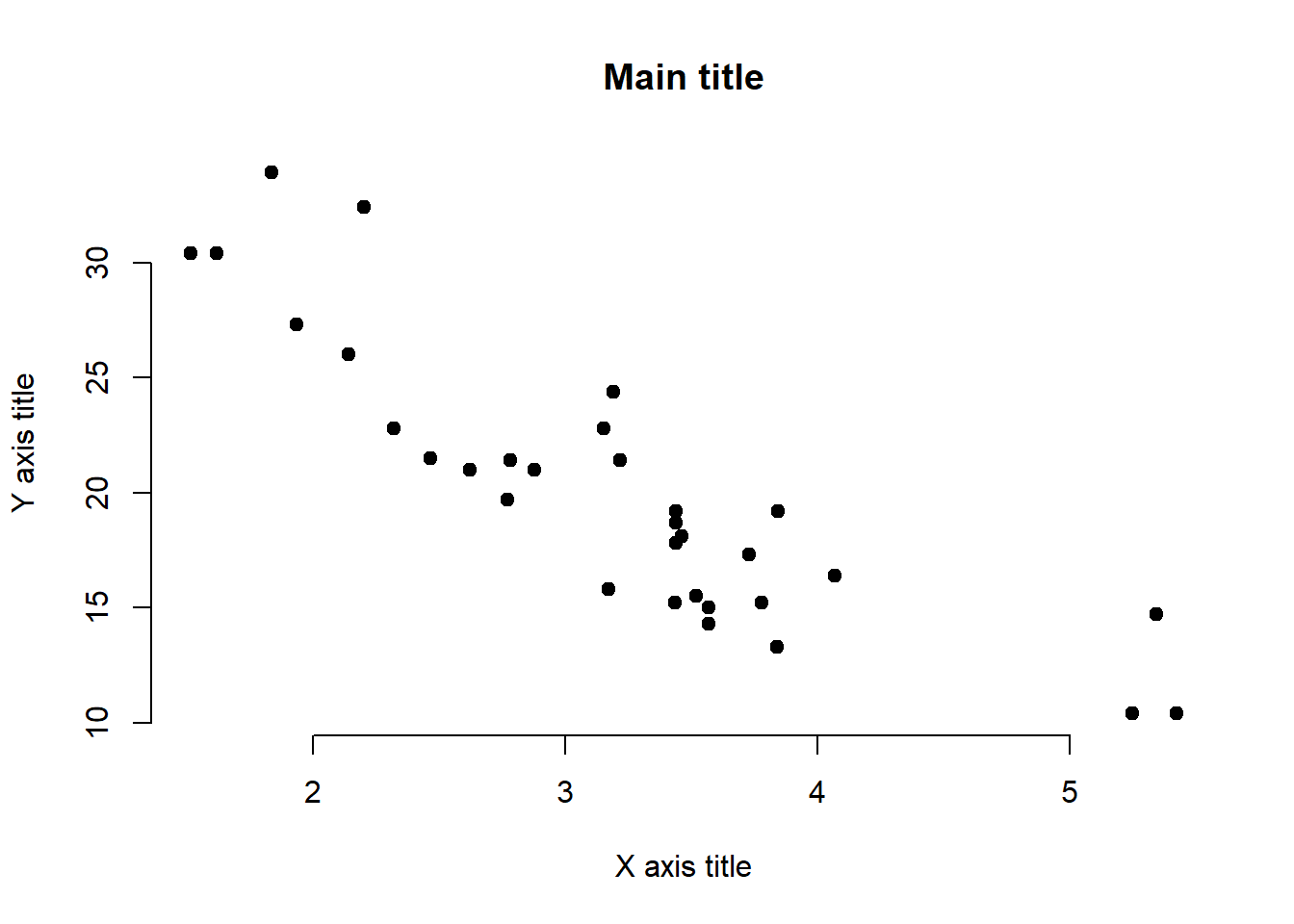# Add regression line
plot(x, y, main = "Main title",
xlab = "X axis title", ylab = "Y axis title",
pch = 19, frame = FALSE)
abline(lm(y ~ x, data = mtcars), col = "blue")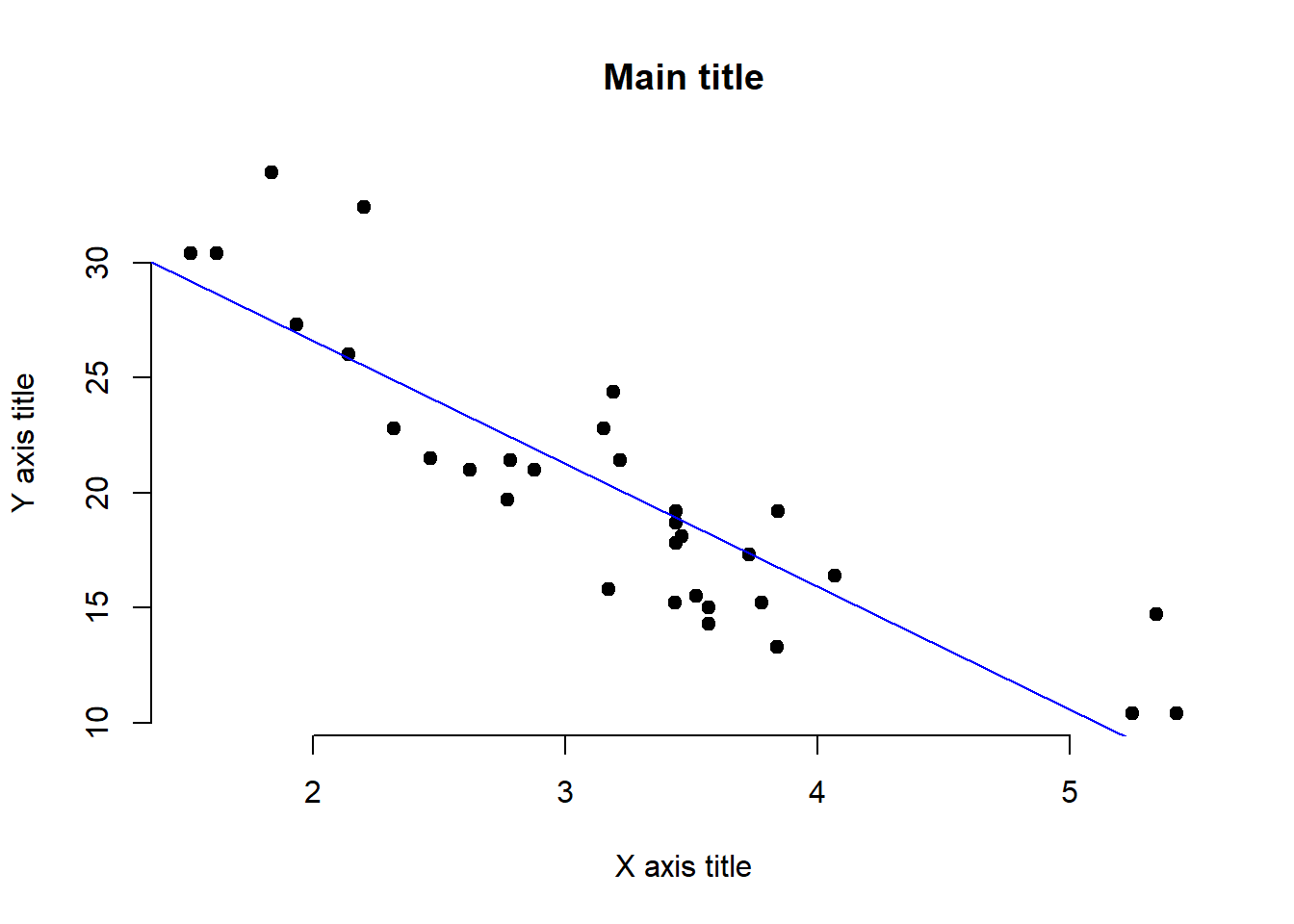# Add loess fit
plot(x, y, main = "Main title",
xlab = "X axis title", ylab = "Y axis title",
pch = 19, frame = FALSE)
lines(lowess(x, y), col = "blue")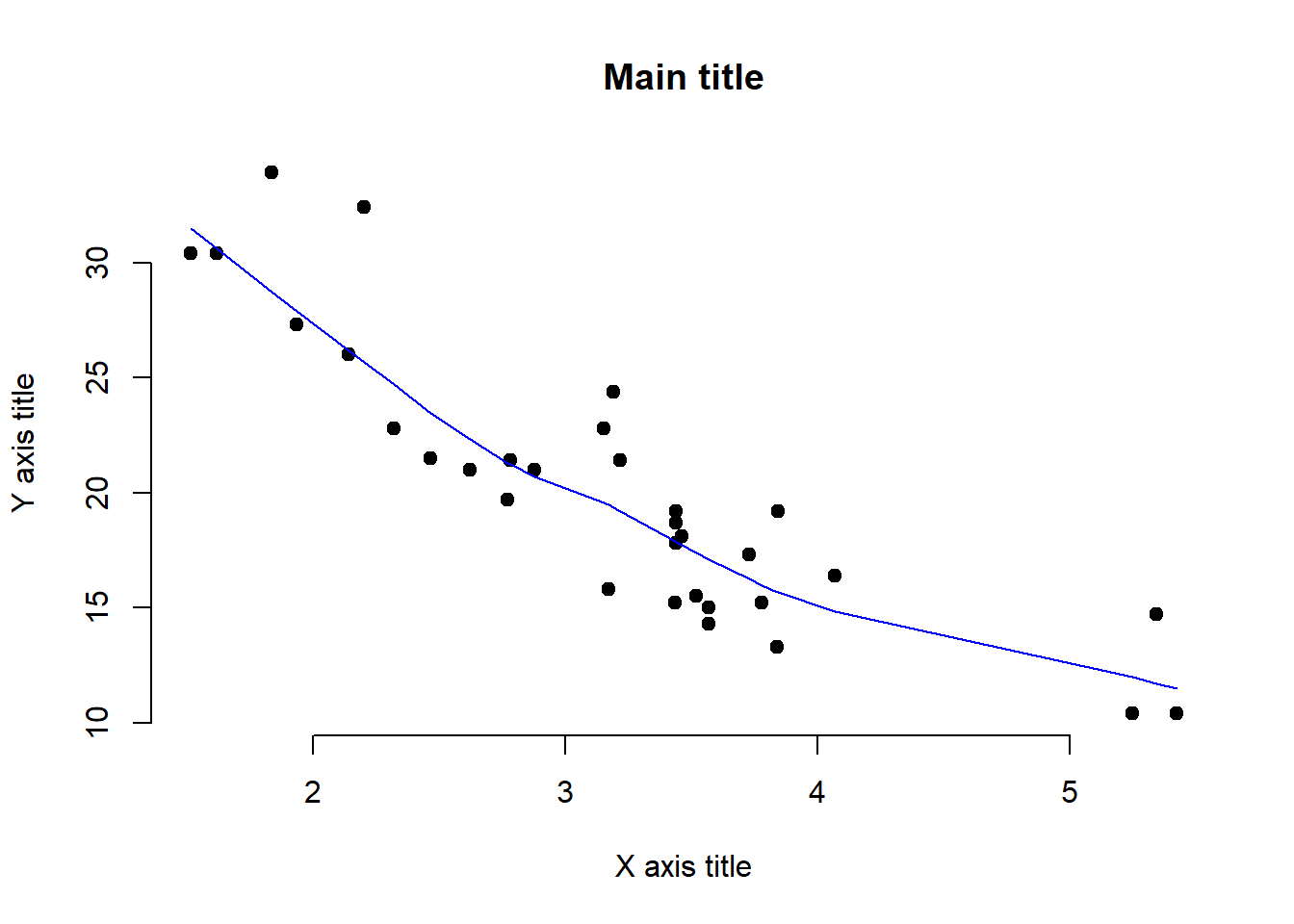Plot Reference plot(x, y = NULL, type = “p”, xlim = NULL, ylim = NULL, log = "“, main = NULL, sub = NULL, xlab = NULL, ylab = NULL, ann = par(”ann"), axes = TRUE, frame.plot = axes, panel.first = NULL, panel.last = NULL, asp = NA, xgap.axis = NA, ygap.axis = NA, …) Arguments x, y the x and y arguments provide the x and y coordinates for the plot. Any reasonable way of defining the coordinates is acceptable. See the function xy.coords for details. If supplied separately, they must be of the same length.

type 1-character string giving the type of plot desired. The following values are possible, for details, see plot: “p” for points, “l” for lines, “b” for both points and lines, “c” for empty points joined by lines, “o” for overplotted points and lines, “s” and “S” for stair steps and “h” for histogram-like vertical lines. Finally, “n” does not produce any points or lines.

xlim the x limits (x1, x2) of the plot. Note that x1 > x2 is allowed and leads to a ‘reversed axis’.

The default value, NULL, indicates that the range of the finite values to be plotted should be used.

ylim the y limits of the plot.

log a character string which contains “x” if the x axis is to be logarithmic, “y” if the y axis is to be logarithmic and “xy” or “yx” if both axes are to be logarithmic.

sub a sub title for the plot.

xlab a label for the x axis, defaults to a description of x.

ylab a label for the y axis, defaults to a description of y.

ann a logical value indicating whether the default annotation (title and x and y axis labels) should appear on the plot.

axes a logical value indicating whether both axes should be drawn on the plot. Use graphical parameter “xaxt” or “yaxt” to suppress just one of the axes.

frame.plot a logical indicating whether a box should be drawn around the plot.

panel.first an ‘expression’ to be evaluated after the plot axes are set up but before any plotting takes place. This can be useful for drawing background grids or scatterplot smooths. Note that this works by lazy evaluation: passing this argument from other plot methods may well not work since it may be evaluated too early.

panel.last an expression to be evaluated after plotting has taken place but before the axes, title and box are added. See the comments about panel.first.

asp the y/x aspect ratio, see plot.window.

xgap.axis, ygap.axis the x/y axis gap factors, passed as gap.axis to the two axis() calls (when axes is true, as per default).

… other graphical parameters (see par and section ‘Details’ below).

Details Commonly used graphical parameters are:

col The colors for lines and points. Multiple colors can be specified so that each point can be given its own color. If there are fewer colors than points they are recycled in the standard fashion. Lines will all be plotted in the first colour specified.

bg a vector of background colors for open plot symbols, see points. Note: this is not the same setting as par(“bg”).

pch a vector of plotting characters or symbols: see points.

cex a numerical vector giving the amount by which plotting characters and symbols should be scaled relative to the default. This works as a multiple of par(“cex”). NULL and NA are equivalent to 1.0. Note that this does not affect annotation: see below.

lty a vector of line types, see par.

cex.main, col.lab, font.sub, etc settings for main- and sub-title and axis annotation, see title and par.

lwd a vector of line widths, see par.

Speed <- cars$speed Distance <- cars$dist
plot(Speed, Distance, panel.first = grid(8, 8),
pch = 0, cex = 1.2, col = "blue")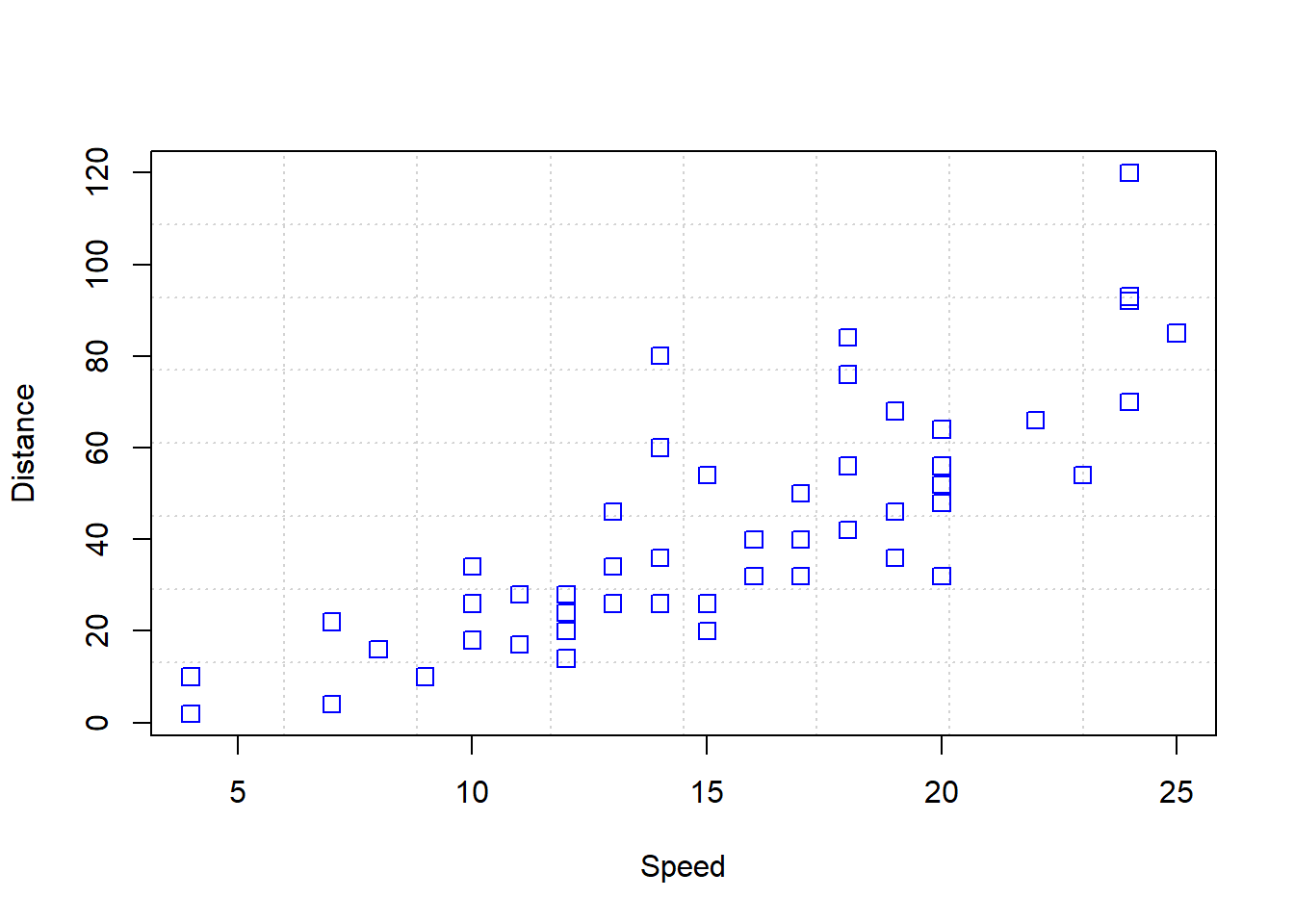plot(Speed, Distance,
panel.first = lines(stats::lowess(Speed, Distance), lty = "dashed"),
pch = 0, cex = 1.2, col = "blue")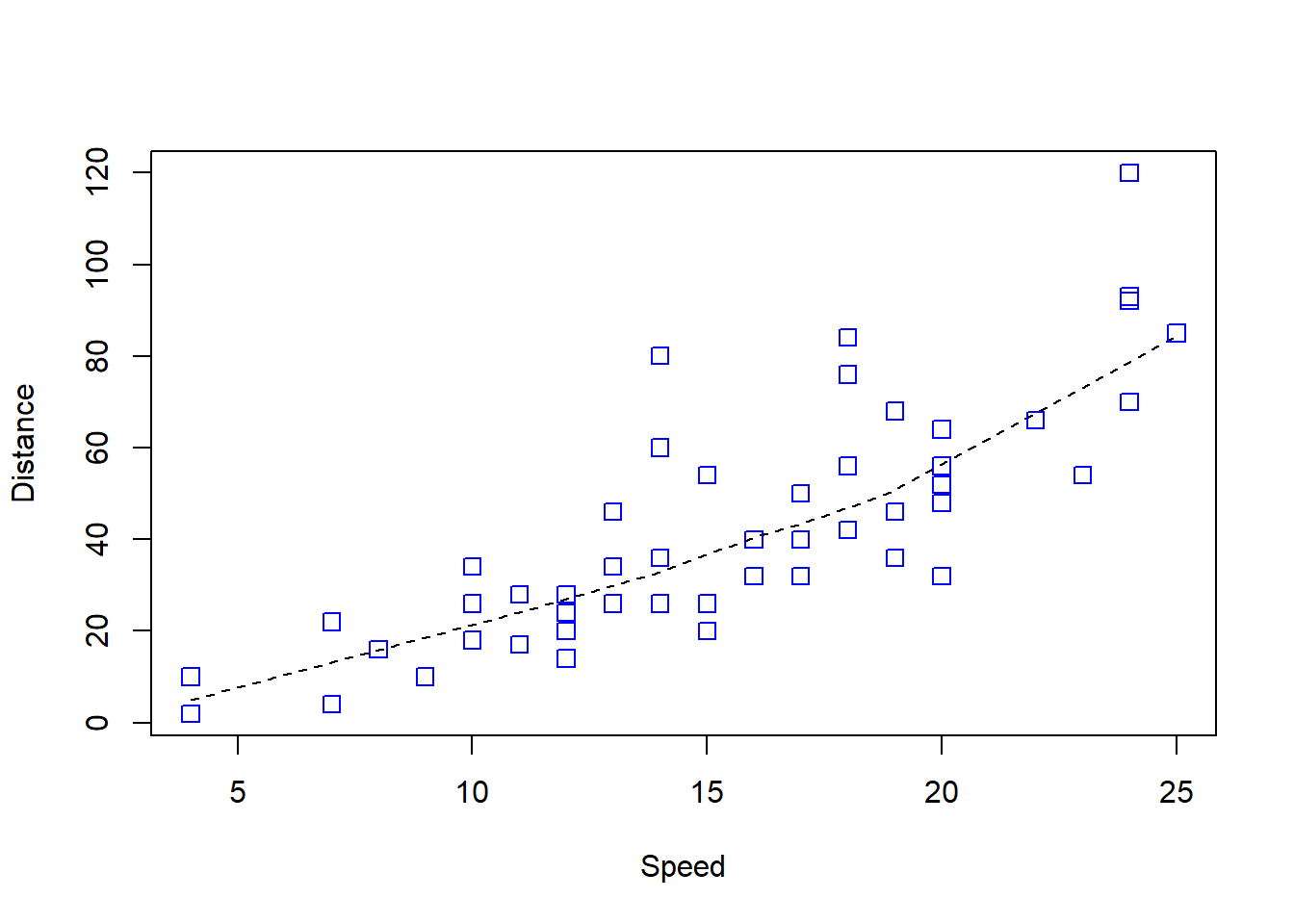## Show the different plot types
x <- 0:12
y <- sin(pi/5 * x)
op <- par(mfrow = c(3,3), mar = .1+ c(2,2,3,1))
for (tp in c("p","l","b",  "c","o","h",  "s","S","n")) {
plot(y ~ x, type = tp, main = paste0("plot(*, type = \"", tp, "\")"))
if(tp == "S") {
lines(x, y, type = "s", col = "red", lty = 2)
mtext("lines(*, type = \"s\", ...)", col = "red", cex = 0.8)
}
}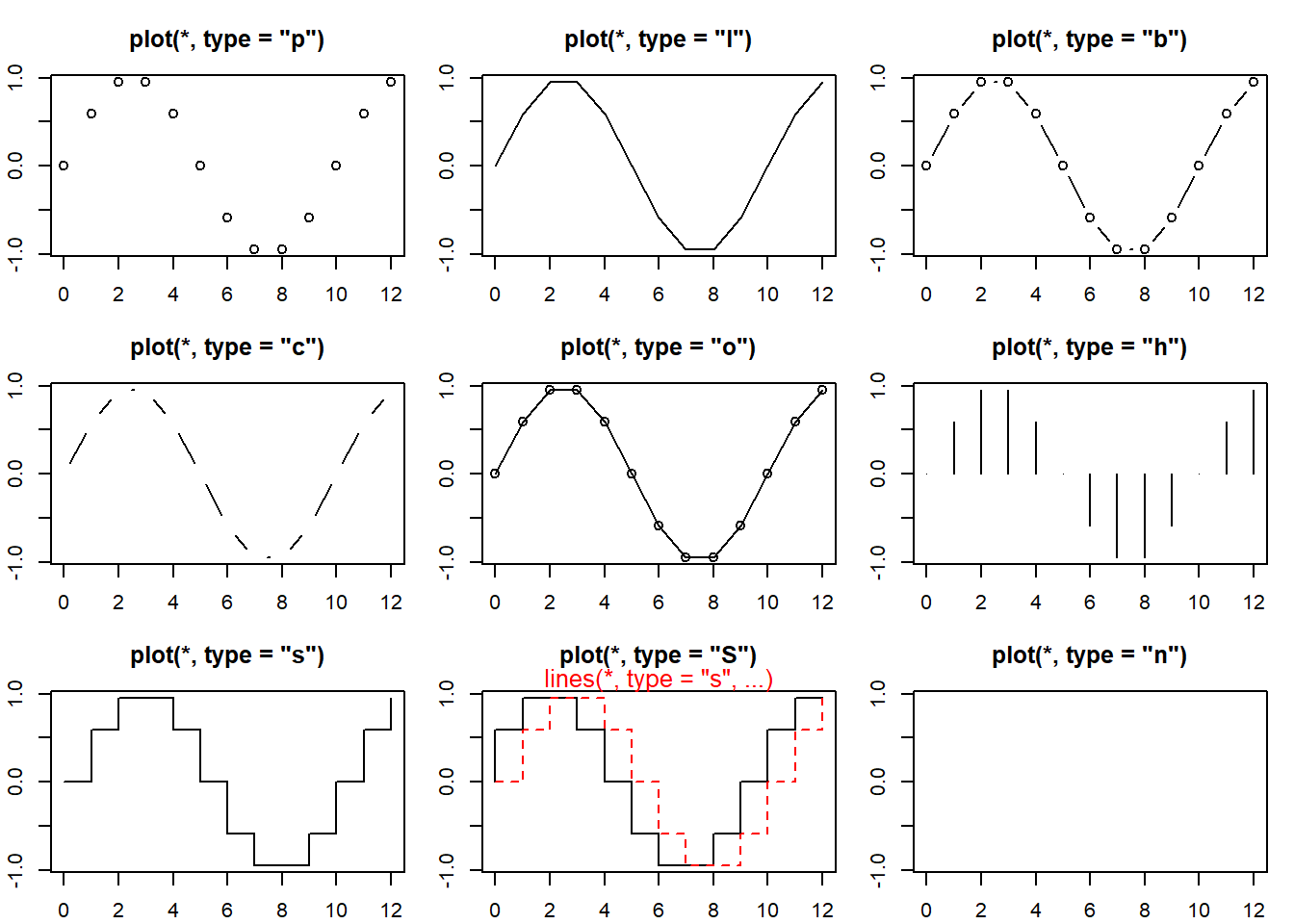par(op)
##--- Log-Log Plot  with  custom axes
lx <- seq(1, 5, length = 41)
yl <- expression(e^{-frac(1,2) * {log(x)}^2})
y <- exp(-.5*lx^2)
op <- par(mfrow = c(2,1), mar = par("mar")-c(1,0,2,0), mgp = c(2, .7, 0))
plot(10^lx, y, log = "xy", type = "l", col = "purple",
main = "Log-Log plot", ylab = yl, xlab = "x")
plot(10^lx, y, log = "xy", type = "o", pch = ".", col = "forestgreen",
main = "Log-Log plot with custom axes", ylab = yl, xlab = "x",
axes = FALSE, frame.plot = TRUE)
my.at <- 10^(1:5)
axis(1, at = my.at, labels = formatC(my.at, format = "fg"))
e.y <- -5:-1 ; at.y <- 10^e.y
axis(2, at = at.y, col.axis = "red", las = 1,
labels = as.expression(lapply(e.y, function(E) bquote(10^.(E)))))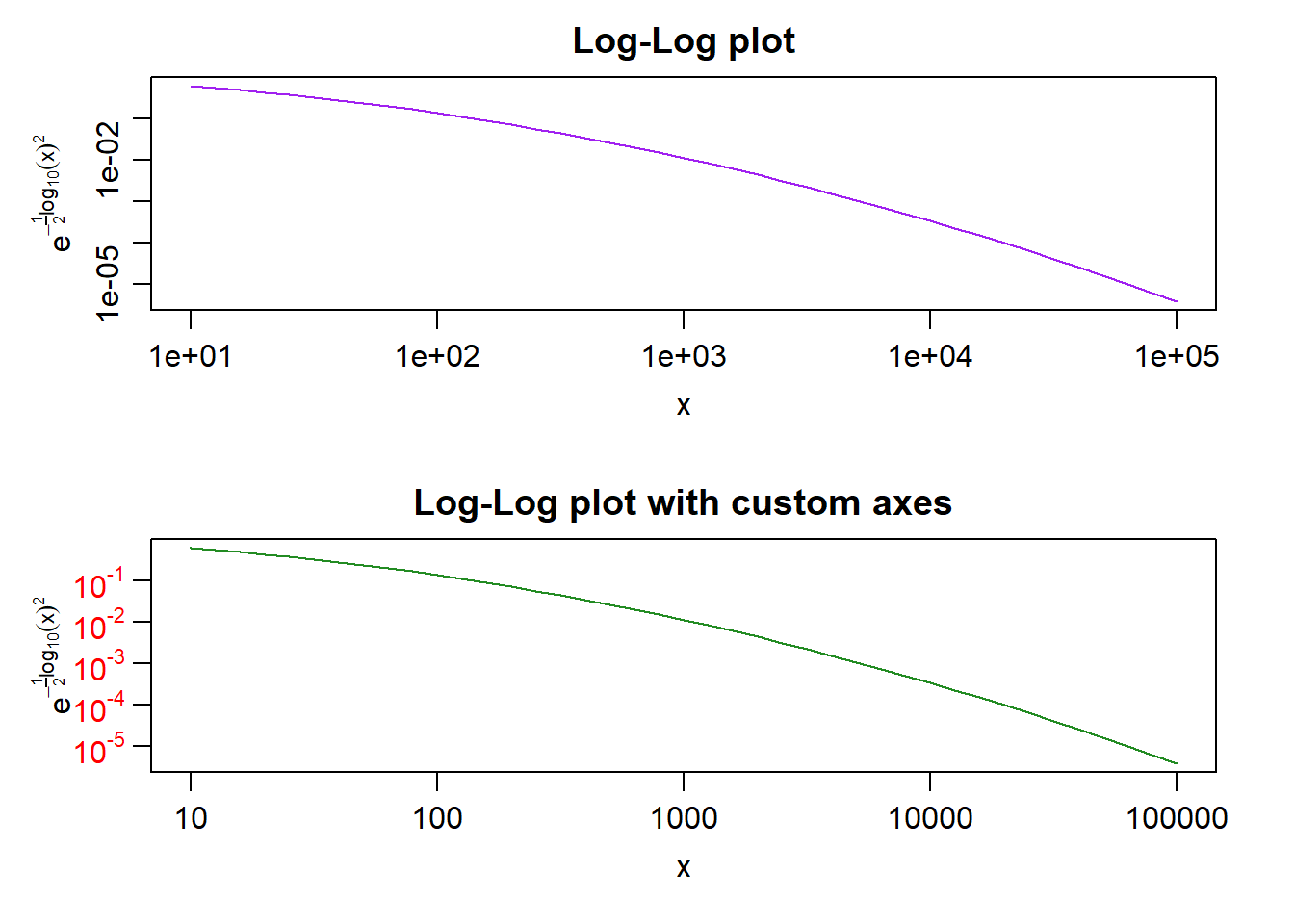par(op)